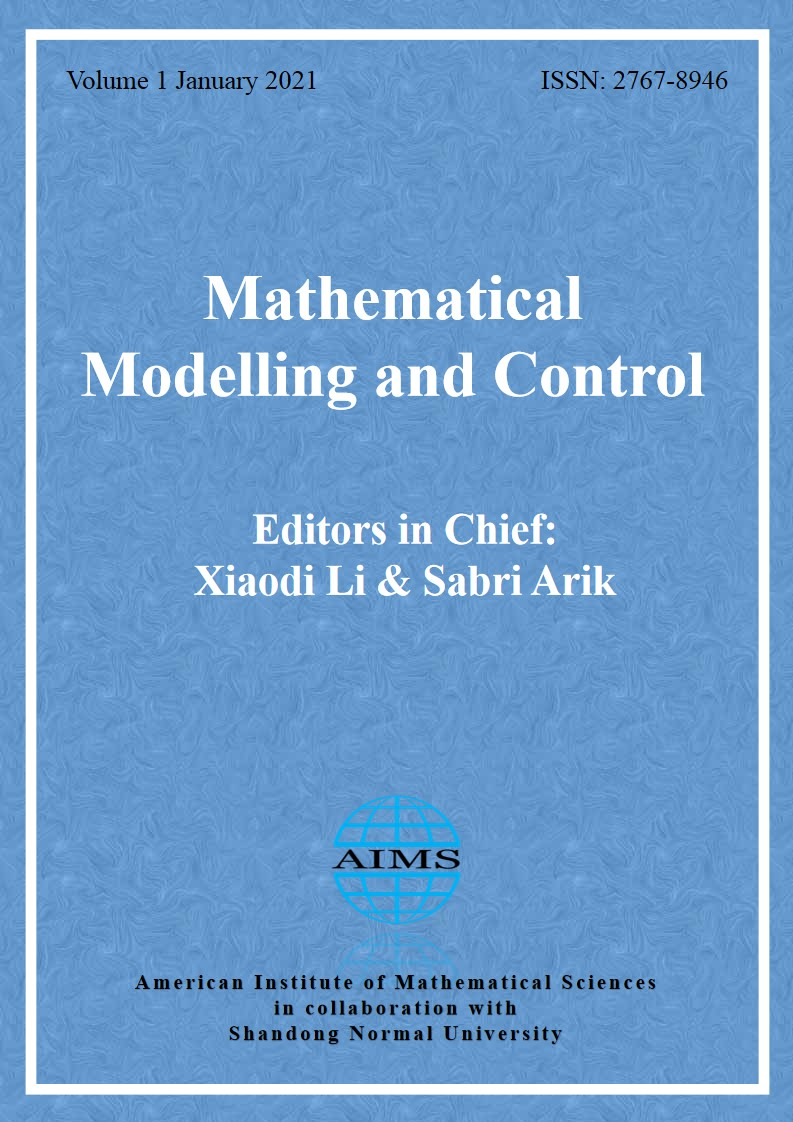### Mathematical Modelling and Control

2021, Issue 2: 79-89. doi: 10.3934/mmc.2021006
Research article

# SVD-Krylov based techniques for structure-preserving reduced order modelling of second-order systems

• Correction on: Mathematical Modelling and Control 1: 134-135
• Received: 21 March 2021 Accepted: 16 June 2021 Published: 22 June 2021
• We introduce an efficient structure-preserving model-order reduction technique for the large-scale second-order linear dynamical systems by imposing two-sided projection matrices. The projectors are formed based on the features of the singular value decomposition (SVD) and Krylov-based model-order reduction methods. The left projector is constructed by utilizing the concept of the observability Gramian of the systems and the right one is made by following the notion of the interpolation-based technique iterative rational Krylov algorithm (IRKA). It is well-known that the proficient model-order reduction technique IRKA cannot ensure system stability, and the Gramian based methods are computationally expensive. Another issue is preserving the second-order structure in the reduced-order model. The structure-preserving model-order reduction provides a more exact approximation to the original model with maintaining some significant physical properties. In terms of these perspectives, the proposed method can perform better by preserving the second-order structure and stability of the system with minimized $\mathcal{H}_2$-norm. Several model examples are presented that illustrated the capability and accuracy of the introducing technique.

Citation: Md. Motlubar Rahman, Mahtab Uddin, M. Monir Uddin, L. S. Andallah. SVD-Krylov based techniques for structure-preserving reduced order modelling of second-order systems[J]. Mathematical Modelling and Control, 2021, 1(2): 79-89. doi: 10.3934/mmc.2021006

### Related Papers:

• We introduce an efficient structure-preserving model-order reduction technique for the large-scale second-order linear dynamical systems by imposing two-sided projection matrices. The projectors are formed based on the features of the singular value decomposition (SVD) and Krylov-based model-order reduction methods. The left projector is constructed by utilizing the concept of the observability Gramian of the systems and the right one is made by following the notion of the interpolation-based technique iterative rational Krylov algorithm (IRKA). It is well-known that the proficient model-order reduction technique IRKA cannot ensure system stability, and the Gramian based methods are computationally expensive. Another issue is preserving the second-order structure in the reduced-order model. The structure-preserving model-order reduction provides a more exact approximation to the original model with maintaining some significant physical properties. In terms of these perspectives, the proposed method can perform better by preserving the second-order structure and stability of the system with minimized $\mathcal{H}_2$-norm. Several model examples are presented that illustrated the capability and accuracy of the introducing technique.###### 通讯作者: 陈斌, bchen63@163.com
• 1.

沈阳化工大学材料科学与工程学院 沈阳 1101421.6

Article outline

## Other Articles By Authors

• On This SiteDownLoad:  Full-Size Img  PowerPoint